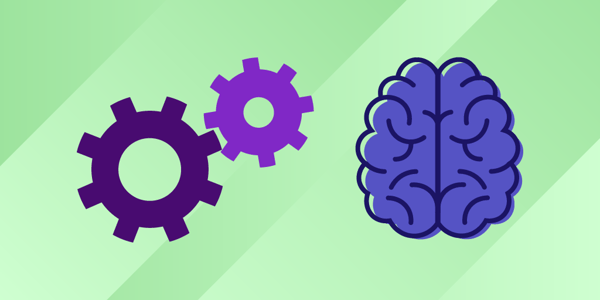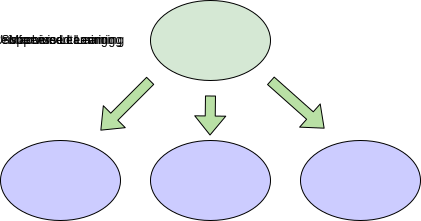# Scikit-learn cheat sheet: methods for classification & regression

Oct 19, 2020 - 6 min read
Aman AnandMachine Learning is a fast-growing technology in today’s world. Machine learning is already integrated into our daily lives with tools like face recognition, home assistants, resume scanners, and self-driving cars.

Scikit-learn is the most popular Python library for performing classification, regression, and clustering algorithms. It is an essential part of other Python data science libraries like matplotlib, NumPy (for graphs and visualization), and SciPy (for mathematics).

In our last article on Scikit-learn, we introduced the basics of this library alongside the most common operations. Today, we take our Scikit-learn knowledge one step further and teach you how to perform classification and regression, followed by the 10 most popular methods for each.

Today, we will cover:

## Refresher on Machine Learning

Machine Learning is teaching the computer to perform and learn tasks without being explicitly coded. This means that the system possesses a certain degree of decision-making capabilities. Machine Learning can be divided into three major categories:

• Supervised Learning
• Unsupervised Learning
• Reinforcement LearningTypes of Machine Learning

### Supervised Learning

In this ML model, our system learns under the supervision of a teacher. The model has both a known input and output used for training. The teacher knows the output during the training process and trains the model to reduce the error in prediction. The two major types of supervised learning methods are Classification and Regression.

### Unsupervised Learning

Unsupervised Learning refers to models where there is no supervisor for the learning process. The model uses just input for training. The output is learned from the inputs only. The major type of unsupervised learning is Clustering, in which we cluster similar things together to find patterns in unlabeled datasets.

### Reinforcement Learning

Reinforcement Learning refers to models that learn to make decisions based on rewards or punishments and tries to maximize the rewards with correct answers. Reinforcement learning is commonly used for gaming algorithms or robotics, where the robot learns by performing tasks and receiving feedback.

In this post I am going to explain the two major methods of Supervised Learning:

• Classification: In Classification, the output is discrete data. In simpler words, this means that we are going to categorize data based on certain features. For example, differentiating between Apples and Oranges based on their shapes, color, texture, etc. In this example shape, color and texture are known as features, and the output is “Apple” or “Orange”, which are known as Classes. Since the output is known as classes, the method is called Classification.
• Regression: In Regression, the output is continuous data. In this method, we predict the trends of training data based on the features. The result does not belong to a certain category or class, but it gives a numeric output that is a real number. For example, predicting House Prices is based on certain features like size of the house, location of the house, and no. of floors, etc.

## How to implement classification and regression

Python provides a lot of tools for implementing Classification and Regression. The most popular open-source Python data science library is scikit-learn. Let’s learn how to use scikit-learn to perform Classification and Regression in simple terms.

The basic steps of supervised machine learning include:

• Split the dataset into training and test set
• Train the model
• Evaluate the model

#Numpy deals with large arrays and linear algebra
import numpy as np
# Library for data manipulation and analysis
import pandas as pd

# Metrics for Evaluation of model Accuracy and F1-score
from sklearn.metrics  import f1_score,accuracy_score

#Importing the Decision Tree from scikit-learn library
from sklearn.tree import DecisionTreeClassifier

# For splitting of data into train and test set
from sklearn.model_selection import train_test_split


train=pd.read_csv("/input/hcirs-ctf/train.csv")
# from path given and stores in the variable named train
# the data type of train is DataFrame


### Splitting into Train & Test set

#first we split our data into input and output
# y is the output and is stored in "Class" column of dataframe
# X contains the other columns and are features or input
y = train.Class
train.drop(['Class'], axis=1, inplace=True)
X = train

# Now we split the dataset in train and test part
# here the train set is 75% and test set is 25%
X_train, X_test, y_train, y_test = train_test_split(X, y, test_size=0.25, random_state=2)


### Training the model

# Training the model is as simple as this
# Use the function imported above and apply fit() on it
DT= DecisionTreeClassifier()
DT.fit(X_train,y_train)


### Evaluating the model

# We use the predict() on the model to predict the output
pred=DT.predict(X_test)

# for classification we use accuracy and F1 score
print(accuracy_score(y_test,pred))
print(f1_score(y_test,pred))

# for regression we use R2 score and MAE(mean absolute error)
# all other steps will be same as classification as shown above
from sklearn.metrics import mean_absolute_error
from sklearn.metrics import r2_score
print(mean_absolute_error(y_test,pred))
print(mean_absolute_error(y_test,pred))


Now that we know the basic steps for Classification and Regression, let’s learn about the top methods for Classification and Regression that you can use in your ML systems. These methods will simplify your ML programming.

Note: Import these methods to use in place of the DecisionTreeClassifier().

## 10 popular classification methods

### Logistic Regression

from sklearn.linear_model import LogisticRegression


### Support Vector Machine

from sklearn.svm import SVC


### Naive Bayes (Gaussian, Multinomial)

from sklearn.naive_bayes import GaussianNB
from sklearn.naive_bayes import MultinomialNB


from sklearn.linear_model import SGDClassifier


### KNN (k-nearest neighbor)

from sklearn.neighbors import KNeighborsClassifier


### Decision Tree

from sklearn.tree import DecisionTreeClassifier


### Random Forest

from sklearn.ensemble import RandomForestClassifier


from sklearn.ensemble import GradientBoostingClassifier


### LGBM Classifier

from lightgbm import LGBMClassifier


### XGBoost Classifier

from xgboost.sklearn import XGBClassifier


## 10 popular regression methods

### Linear Regression

from sklearn.linear_model import LinearRegression


### LGBM Regressor

from lightgbm import LGBMRegressor


### XGBoost Regressor

from xgboost.sklearn import XGBRegressor


### CatBoost Regressor

from catboost import CatBoostRegressor


from sklearn.linear_model import SGDRegressor


### Kernel Ridge Regression

from sklearn.kernel_ridge import KernelRidge


### Elastic Net Regression

from sklearn.linear_model import ElasticNet


### Bayesian Ridge Regression

from sklearn.linear_model import BayesianRidge


from sklearn.ensemble import GradientBoostingRegressor


### Support Vector Machine

from sklearn.svm import SVR


## What to learn next

I hope this short tutorial and cheat sheet is helpful for your scikit-learn journey. These methods will make your data scientist journey much smoother and simpler as you continue to learn these powerful tools. There is still a lot to learn about Scikit-learn and the other Python ML libraries.

As you continue your Scikit-learn journey, here are the next algorithms and topics to learn:

• Support Vector machine
• Random Forest
• Cross-validation techniques
• grid_search
• fit_transform
• n_clusters
• n_neighbors
• sklearn.grid

To advance your scikit-learn journey, Educative has created the course Hands-on Machine Learning with Scikit-Learn. With in-depth explanations of all the Scikit-learn basics and popular ML algorithms, this course offers everything you need in one place. By the end, you’ll know how and when to use each machine learning algorithm and will have the Scikit skills to stand out to any interviewer.

Happy learning!